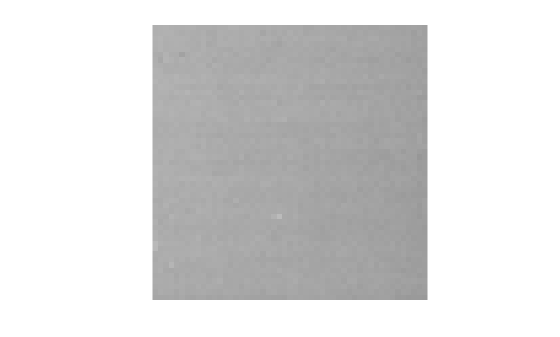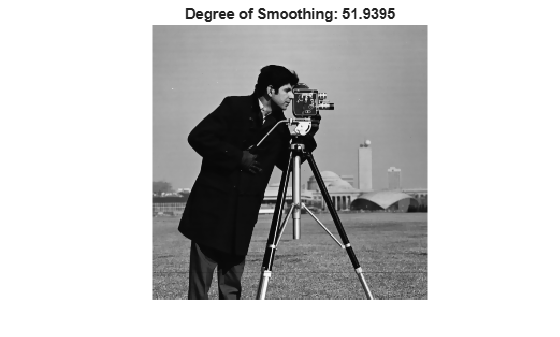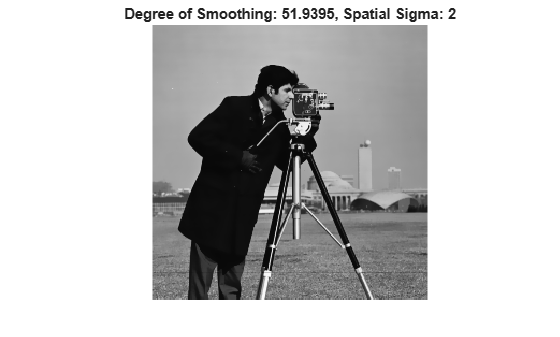# imbilatfilt

Bilateral filtering of images with Gaussian kernels

## Syntax

``J = imbilatfilt(I)``
``J = imbilatfilt(I,degreeOfSmoothing)``
``J = imbilatfilt(I,degreeOfSmoothing,spatialSigma)``
``J = imbilatfilt(___,Name,Value)``

## Description

````J = imbilatfilt(I)` applies an edge-preserving Gaussian bilateral filter to the grayscale or RGB image, `I`.```

example

````J = imbilatfilt(I,degreeOfSmoothing)` specifies the amount of smoothing. When `degreeOfSmoothing` is a small value, `imbilatfilt` smooths neighborhoods with small variance (uniform areas) but does not smooth neighborhoods with large variance, such as strong edges. When the value of `degreeOfSmoothing` increases, `imbilatfilt` smooths both uniform areas and neighborhoods with larger variance.```
````J = imbilatfilt(I,degreeOfSmoothing,spatialSigma)` also specifies the standard deviation, `spatialSigma`, of the spatial Gaussian smoothing kernel. Larger values of `spatialSigma` increase the contribution of more distant neighboring pixels, effectively increasing the neighborhood size.```
````J = imbilatfilt(___,Name,Value)` uses name-value pairs to change the behavior of the bilateral filter.```

## Examples

collapse all

Read and display a grayscale image. Observe the horizontal striation artifact in the sky region.

```I = imread('cameraman.tif'); imshow(I)```Inspect a patch of the image from the sky region. Compute the variance of the patch, which approximates the variance of the noise.

```patch = imcrop(I,[170, 35, 50 50]); imshow(patch)````patchVar = std2(patch)^2;`

Filter the image using bilateral filtering. Set the degree of smoothing to be larger than the variance of the noise.

```DoS = 2*patchVar; J = imbilatfilt(I,DoS); imshow(J) title(['Degree of Smoothing: ',num2str(DoS)])```The striation artifact is reduced, but not eliminated. To improve the smoothing, increase the value of `spatialSigma` to `2` so that distant neighboring pixels contribute more to the Gaussian smoothing kernel. This effectively increases the spatial extent of the bilateral filter.

```K = imbilatfilt(I,DoS,2); imshow(K) title(['Degree of Smoothing: ',num2str(DoS),', Spatial Sigma: 2'])```The striation artifact in the sky is successfully removed. The sharpness of strong edges such as the silhouette of the man, and textured regions such as the grass in the foreground of the image, have been preserved.

```imRGB = imread('coloredChips.png'); imshow(imRGB)```Convert the image to the L*a*b colorspace, so that the bilateral filter smooths perceptually similar colors.

`imLAB = rgb2lab(imRGB);`

Extract an L*a*b patch that contains no sharp edges. Compute the variance in the Euclidean distance from the origin, in the L*a*b space.

```patch = imcrop(imLAB,[34,71,60,55]); patchSq = patch.^2; edist = sqrt(sum(patchSq,3)); patchVar = std2(edist).^2;```

Filter the image in the L*a*b* color space using bilateral filtering. Set the `DegreeOfSmoothing` value to be higher than the variance of the patch.

```DoS = 2*patchVar; smoothedLAB = imbilatfilt(imLAB,DoS);```

Convert the image back to the RGB color space, and display the smoothed image.

```smoothedRBG = lab2rgb(smoothedLAB,'Out','uint8'); montage({imRGB,smoothedRBG}) title(['Original Image vs. Filtered Image with Degree of Smoothing: ',num2str(DoS)])```The colors of the chips and black pen appear more uniform, but the horizontal grains in the table are still visible. Increase the spatial extent of the filter so that the effective neighborhood of the filter spans the space between the horizontal grains (this distance is approximately seven pixels). Also increase the `DegreeOfSmoothing` to smooth these regions more aggressively.

```DoS2 = 4*patchVar; smoothedLAB2 = imbilatfilt(imLAB,DoS2,7); smoothedRBG2 = lab2rgb(smoothedLAB2,'Out','uint8'); montage({imRGB,smoothedRBG2}) title(['Original Image vs. Filtered Image with Degree of Smoothing: ',num2str(DoS),' and Spatial Sigma: 7'])```The color of the wooden table is more uniform with the larger neighborhood and larger degree of smoothing. The edge sharpness of the chips and pen is preserved.

## Input Arguments

collapse all

Image to filter, specified as a 2-D grayscale image of size m-by-n or a 2-D color image of size m-by-n-by-3.

Data Types: `single` | `double` | `int8` | `int16` | `int32` | `uint8` | `uint16` | `uint32`

Degree of smoothing, specified as a positive number. The default value of `degreeOfSmoothing` depends on the data type of image `I`, and is calculated as `0.01*diff(getrangefromclass(I)).^2`. For example, the default degree of smoothing is `650.25` for images of data type `uint8`, and the default is `0.01` for images of data type `double` with pixel values in the range [0, 1].

Standard deviation of spatial Gaussian smoothing kernel, specified as a positive number.

### Name-Value Pair Arguments

Specify optional comma-separated pairs of `Name,Value` arguments. `Name` is the argument name and `Value` is the corresponding value. `Name` must appear inside quotes. You can specify several name and value pair arguments in any order as `Name1,Value1,...,NameN,ValueN`.

Example: `imbilatfilt(I,'NeighborhoodSize',7)` performs bilateral filtering on image `I` using a 7-by-7 pixel neighborhood

Neighborhood size, specified as the comma-separated pair consisting of `'NeighborhoodSize'` and an odd-valued positive integer. By default, the neighborhood size is `2*ceil(2*SpatialSigma)+1` pixels

Example: `'NeighborhoodSize',7`

Data Types: `single` | `double` | `int8` | `int16` | `int32` | `int64` | `uint8` | `uint16` | `uint32` | `uint64`

Padding, specified as the comma-separated pair consisting of `'Padding'` and one of these values.

ValueDescription
`'replicate'`Input array values outside the bounds of the array are assumed to equal the nearest array border value.
`'symmetric'`

Input array values outside the bounds of the array are computed by mirror-reflecting the array across the array border.

numeric scalar, xInput image values outside the bounds of the image are assigned the value x.

Example: `'Padding','symmetric'`

Example: `'Padding',128`

Data Types: `single` | `double` | `int8` | `int16` | `int32` | `int64` | `uint8` | `uint16` | `uint32` | `uint64` | `char` | `string`

## Output Arguments

collapse all

Filtered image, returned as a numeric array of the same size and data type as the input image, `I`.

## Tips

• The value of `degreeOfSmoothing` corresponds to the variance of the Range Gaussian kernel of the bilateral filter . The Range Gaussian is applied on the Euclidean distance of a pixel value from the values of its neighbors.

• To smooth perceptually close colors of an RGB image, convert the image to the CIE L*a*b space using `rgb2lab` before applying the bilateral filter. To view the results, convert the filtered image to RGB using `lab2rgb`.

• Increasing `spatialSigma` increases `NeighborhoodSize`, which increases the filter execution time. You can specify a smaller `NeighborhoodSize` to trade accuracy for faster execution time.

 Tomasi, C., and R. Manduchi. "Bilateral Filtering for Gray and Color Images". Proceedings of the 1998 IEEE® International Conference on Computer Vision. Bombay, India. Jan 1998, pp. 836–846.